Download PresentationHypotheses and Trend Analysis

# Hypotheses and Trend Analysis - PowerPoint PPT Presentation

Hypotheses and Trend Analysis. W rite a hypothesis (& science reason) for the following:. IV DV 1. temperature °C Solubility ( solute g/100 g of water) 2. mass (g) of box force (N) to overcome frictionI am the owner, or an agent authorized to act on behalf of the owner, of the copyrighted work described.
Download Presentation## Hypotheses and Trend Analysis

An Image/Link below is provided (as is) to download presentation

Download Policy: Content on the Website is provided to you AS IS for your information and personal use and may not be sold / licensed / shared on other websites without getting consent from its author.While downloading, if for some reason you are not able to download a presentation, the publisher may have deleted the file from their server.

- - - - - - - - - - - - - - - - - - - - - - - - - - E N D - - - - - - - - - - - - - - - - - - - - - - - - - -
Presentation Transcript### Hypotheses and Trend Analysis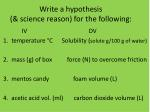Write a hypothesis (& science reason) for the following:

IV DV

• 1. temperature °C Solubility (solute g/100 g of water)
• 2. mass (g) of box force (N) to overcome friction
• 3. mentos candy foam volume (L)
• 4. acetic acid vol. (ml) carbon dioxide volume (L)Hypothesis Wording for Quantity
• You make a prediction and then justify it with a science concept.
• If (your IV increases), then (your DV response to the change).
• Simply worded – not If (IV), then (IV change that will most affect DV).
• This will happen because … use the science to justify.
• Compare your hypothesis to the trend statement: As (your IV) increases, the (DV)… What’s the response to the change? State what the best fit line does.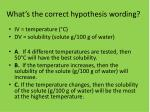What’s the correct hypothesis wording?
• IV = temperature (°C)
• DV = solubility (solute g/100 g of water)
• A. If 4 different temperatures are tested, then 50°C will have the best solubility.
• B. If the temperature increases, then the solubility of the solute (g/100 g of water) will increase.
• C. If the temperature changes, then the solubility of the solute (g/100 g of water) will be the most at the highest temperature.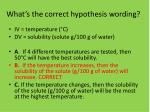What’s the correct hypothesis wording?
• IV = temperature (°C)
• DV = solubility (solute g/100 g of water)
• A. If 4 different temperatures are tested, then 50°C will have the best solubility.
• B. If the temperature increases, then the solubility of the solute (g/100 g of water) will increase. CORRECT
• C. If the temperature changes, then the solubility of the solute (g/100 g of water) will be the most at the highest temperature.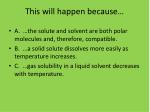This will happen because…
• A. …the solute and solvent are both polar molecules and, therefore, compatible.
• B. …a solid solute dissolves more easily as temperature increases.
• C. …gas solubility in a liquid solvent decreases with temperature.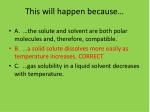This will happen because…
• A. …the solute and solvent are both polar molecules and, therefore, compatible.
• B. …a solid solute dissolves more easily as temperature increases. CORRECT
• C. …gas solubility in a liquid solvent decreases with temperature.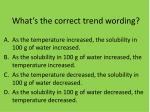### What’s the correct trend wording?

As the temperature increased, the solubility in 100 g of water increased.

As the solubility in 100 g of water increased, the temperature increased.

As the temperature decreased, the solubility in 100 g of water decreased.

As the solubility in 100 g of water decreased, the temperature decreased.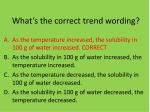### What’s the correct trend wording?

As the temperature increased, the solubility in 100 g of water increased. CORRECT

As the solubility in 100 g of water increased, the temperature increased.

As the temperature decreased, the solubility in 100 g of water decreased.

As the solubility in 100 g of water decreased, the temperature decreased.Don’t forget the data to support your trend statement.
• At 0°C the solubility was 18 g of solute/100 g of water, ……, and at 100°C the solubility was 49 g of solute/100 g of water. The overall % change from 0° to 100°C was 172%.*
• (Use all the data points – I am saving time)
• *[(49-18)÷18] x 100### What’s the correct trend wording?

As the temperature increased, the solubility in 100 g of water increased.

As the temperature increase, nothing happened at low temperatures; then the solubility increased at higher temperatures.

None of the above.### What’s the correct trend wording?

As the temperature increased, the solubility in 100 g of water increased.

As the temperature increase, nothing happened at low temperatures; then the solubility increased at higher temperatures.

None of the above. CORRECT As the temperature increased the solubility of NaCl in 100 g of water only changed from 38 grams to 38.5 grams. The temperature did not significantly affect the solubility.### What’s the correct trend wording?Same data – scale is important.

There is no trend.

As the temperature increased, the solubility of NaCl in 100 g of water did not change.### What’s the correct trend wording?Same data – scale is important.

There is no trend.

As the temperature increased, the solubility of NaCl in 100 g of water did not change. CORRECT

The graph is showing the same data as the previous graph, just with a different scale. The scale on the first graph was such that it skewed the data to look like there was a change when there really wasnt;.Supporting Data
• At 0°C the solubility was 38 g of NaCl /100 g of water, ……, and at 50°C the solubility was 38.5 g /100 g of water. The percent change from 0° to 50°C was only 1.3%*.
• Again, I saved time, but you need to state all the data. Use % change to really show effect (or no effect).
• *[(38.5 – 38) ÷ 38] x 100What’s the correct hypothesis wording?
• IV = mass (g) of box
• DV = force (N) to overcome friction
• A. If 4 different size boxes are tested, then smallest size will need the least amount of force.
• B. If box mass is tested, then the 20 g size will need the most force to overcome friction.
• C. If the box mass (g) increases, then the force (N) to overcome friction will increase.What’s the correct hypothesis wording?
• IV = mass (g) of box
• DV = force (N) to overcome friction
• A. If 4 different size boxes are tested, then smallest size will need the least amount of force.
• B. If box mass is tested, then the 20 g size will need the most force to overcome friction.
• C. If the box mass (g) increases, then the force (N) to overcome friction will increase. CORRECTThis will happen because…
• A. …friction depends on the surface types. Friction increases with surface roughness.
• B. …friction depends on how hard two surfaces press together. Increasing mass of an object increases the force of an object resting on another surface.This will happen because…
• A. …friction depends on the surface types. Friction increases with surface roughness.
• B. …friction depends on how hard two surfaces press together. Increasing mass of an object increases the force of an object resting on another surface. CORRECTCorrect Hypothesis Wording
• Compare your wording.
• What did you do correct?
• Is the wording simple?
• What might the trend look be? Follow the template.IV = mentos candy (number)DV = foam volume (L)
• If the number of mint mentos candies in 2 L of Diet Coke increases, the foam volume (L) produced will increase. The candy’s pitted surface increases the surface area exposed to the soda, which chemically react to produce more carbon dioxide; the CO2 gas forcefully escapes from the soda bottle causing foam.IV = acetic acid volume (mL) DV = carbon dioxide volume (mL)
• If the volume (mL) of acetic acid mixed with sodium bicarbonate increases, then the carbon dioxide volume (mL) will increase. Carbon dioxide gas is produced when an acid and carbonate chemically react. Increasing the reactant(s) increases the products of a chemical reaction.These last two slides show research to explain results. Both experiments were examples of chemical reactions – results were because new substances were produce.